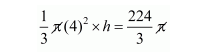# A hollow sphere of internal and external diameters 4 and 8 cm

Question:

A hollow sphere of internal and external diameters 4 and 8 cm respectively is melted into a cone of base diameter 8 cm. Find the height of the cone.

Solution:

Internal radius of hemisphere $r_{1}=\frac{4}{2}=2 \mathrm{~cm}$

External radius of hemisphere $r_{2}=\frac{8}{2}=4 \mathrm{~cm}$

Volume of hollow sphere $=\frac{4}{3} \pi\left(r_{2}^{3}-r_{1}^{3}\right)$

$=\frac{4}{3} \pi(64-8)$

$=\frac{4}{3} \pi \times 56$

$=\frac{224}{3} \pi \mathrm{cm}^{2}$

Since,

The hemisphere melted into a cone of radius r = 4 cm

Let h be height of the cone.

Clearly, the volume of cone = volume of hemisphere$h=\frac{224}{6}$

$h=14$

Thus, the height is 14 cm.